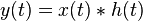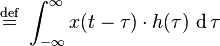# Why is convolution used to represent LTI output?

Nikitin
Hi. If you have a LTI system with an impulse response function ##h(t)## taking in an input ##x(t)##, why does its output ##y(t)## become?

I realize ##y(t)## not only depends on the instantaneous input ##x(t)##, but also on the lingering effects of previous inputs ##x(\tau)## with ##\tau < t##. But how does the convolution integral model this?

•Stephen Tashi

Nikitin
OK I found a mathematical proof on wikipedia. It is coherent, but gives zero physical insight (is this the norm in EE?).

http://en.wikipedia.org/wiki/LTI_system_theory#Continuous-time_systems

However, why does the input ##x(u)## and output ##y(t)## have different domains there? Is it because the range of ##y(t)## is directly dependent on every element in the range of ##x(t)##?

There are LTI systems that do not need to be represented by a convolution. So I think the best intuitive approach is to ask why the most general LTI system is represented by a convolution and why all apparently simpler LTI systems can be regarded as being implemented by a convolution.

Suppose the rule for a system S(t) is "the output y(t) is 4 times the input x(t)".
The notation for this can be confusing, since S can be regarded as function t, but we are also thinking of it as function of x(t).
So , abusing notation, S(t) = S( x(t) ) = 4 x(t).
This system is linear. S( A g(t) + B r(t)) = A S(g(t)) + B S(r(t)).
The output is shift invariant in the sense that S(t-h) = S(x(t-h)) = S applied to input function evalated at t-h..

A slightly more general system is
S(t) = S( x(t)) ) = 4 x(t) + 3 x(t-1) + 2 x(t+1)
This is also linear and shift invariant.

This suggests a more general form for LTI systems is:
$S(t) = \sum_{i=0}^N A_i x(t-h_i)$ where the $A_i$ and $h_i$ are constants.

If the $h_i$ are all distinct numbers then we can define a function $g(h)$ that maps the value $h_i$ to the coefficient $A_i$

Rewriting the above summation in terms of g():

$S(t) = \sum_{i=0}^N g(h_i) x(t - h_i)$

To further generalize, we replace summation by integration

$S(t) = \int_H g(h) x(t-h) dh$ where $H$ is the range of values that $h$ may take.

•Nikitin
2021 Award
OK I found a mathematical proof on wikipedia. It is coherent, but gives zero physical insight (is this the norm in EE?).
Mathematical solutions are deliberately generalised to avoid physical insight as that makes mathematics universally applicable.

So, without using mathematical symbols, why convolution? The important thing is that, being linear, there is no cross-modulation of the signals in the system. All the different frequencies in the response are therefore amplitude and phase independent of each other. The transfer function is then simply the amplitude and phase response of the system to any sinusoidal stimulation. That is not true, as you suggest in the time domain, where all the stimulations and responses are summed in all of time.

•Nikitin
Mike_In_Plano
I have a more intuitive means of appreciating the power of convolutions.
If you think of the LTI as it responds to a short impulse, you have the impulse function.
If you imagine the input signal as a stream of impulses of various magnitudes, you can imagine the system responding to each impulse.
If you graph the response to the first impulse, then graph the response of the second response under it, and continue making graphs down the page, you'll see that the responses are all the same except for magnitude and a slight delay to account for when each impulse happens.

Now, if you make a "totaled" graph at the bottom by adding the values of each graph (y_total(t) = x1(t) + x2(t) +x3(t).... ) above, you get the cumulative effect of the signal over time.
This is essentially what happens when you perform the integration, but this has always seemed "right" to me as it allows you to better imagine the process behind the math.

•Nikitin
The way I look at it, the contribution to the output that input x() makes is "locally linear". At t -h it adds some constant multiple of x(t-h) to the output at t. So you can think of the net output as a sum of the form g(h)x(t-h) where g(h) is a function that tells you what constant to multiply x(t-h) by. (This probably amounts to the "impulse response" view.) If the contribution of the input at t-h was a nonlinear expression like $(x(t-h))^2$ then the output wouldn't be a linear function of the input.

•Nikitin